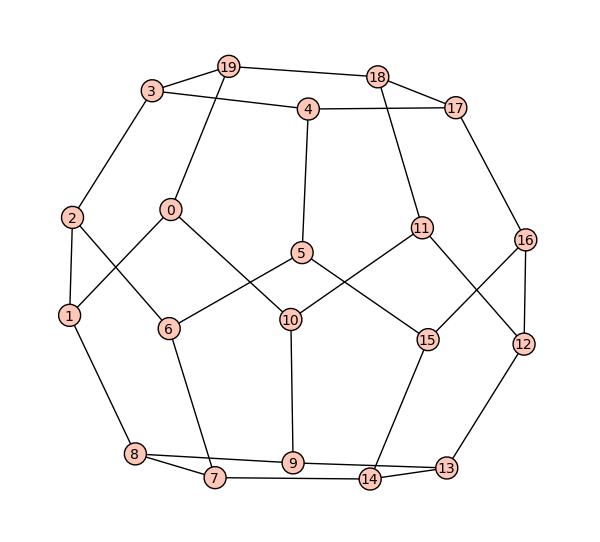MA010 Graph Theory (an online guide)

# Lecture 8: Planarity and drawings of graphs

Outline

•Drawings of graphs, planar graphs
basic properties of planar embeddings of graphs, faces, Jordan curve theorem, Euler's formula, bounding the number of edges of a planar graph
• Characterization of planar graphs
non-planarity of K5 and K3,3, Kuratowski theorem, uniqueness of embeddings
• Colouring maps and planar graphs
the four colour problem, colouring planar graphs by 6 and 5 colours, (the four colour theorem)
• Practical "nice" drawings
how to get aesthetical and understandable (or pleasurable) drawings of a graph, the spring model

Goals

The purpose of this lecture is to give a thorough introduction to planar graphs (those without "crossings" of edges), their basic properties and Kuratowski's characterization of them. From historical perspectives, planar graphs are tied with geometric polyhedra, and their practical importance should be quite obvious -- in many applications, planarity is essential or unavoidable. For historical reasons, we also look at the famous Four Colour Problem, continuing on the colouring topic from the last lecture. Finally, we touch the practical question how to find an aesthetical drawing of a nonplanar graph.Course book  [Invitation to Discrete Mathematics (by Jiří Matoušek, Jaroslav Nešetřil)]:

Study Sections 6.1, 6.2, and 6.3. However, most of the content of 6.1 (about other surfaces) can be left till future Lecture 11.

Remark
Notice that the book first deals with nonplanarity of K5 (which is not so easy to prove in this context) and then introduces Euler's formula, while we first prove Euler's formula and then use it to "easily" prove nonplanarity of K5. The truth is that neither of these statements is actually easy and they both strongly rely on the powerful Jordan curve theorem (which we just briefly mention).